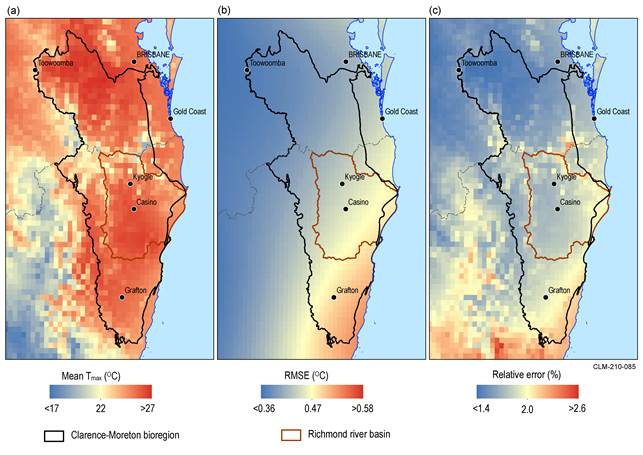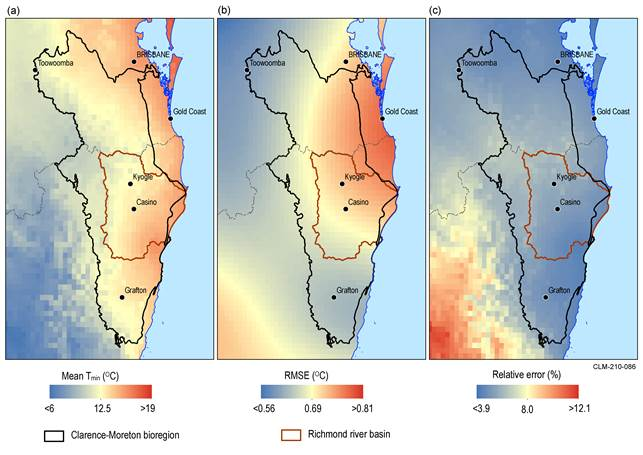# 2.1.1.2 Statistical analysis and interpolation

Page 3 of 77

All geographic data specific to the Clarence-Moreton bioregion were obtained from state or national datasets. This means no statistical analysis or interpolation was performed to generate any of the geographic datasets. However, to characterise errors of the input climate data used for the water balance modelling, some bioregion-specific spatial analysis was performed. This is a useful characterisation to better understand the limitations of the input data. This analysis is outlined in this section.

In addition to generating daily and monthly grids of meteorological variables (P, Tmax and Tmin), the Bureau of Meteorology (Jones et al., 2009) also generate daily and monthly root-mean-square error (RMSE) grids of the same variables. These daily and monthly RMSE grids are a combined measure of the observational error and geostatistical error. The latter is a function of the interpolation algorithm, density of isolated station observations and degree of spatial autocorrelation of the process(es) driving the spatial variance captured in the data being interpolated.

To characterise errors of the input climate data the long-term (from January 1980 to December 2009) monthly mean values for P, Tmax and Tmin were calculated. Also calculated were the long-term monthly RMSE mean values for the same variables for the same time period. Relative error, expressed as a percent, was calculated by dividing the monthly RMSE mean grid by the monthly mean grids (i.e. RMSE grid/mean grid for each meteorological variable).

The spatially-averaged long-term monthly mean P for the Clarence-Moreton bioregion is 110 mm/month, and the associated P RMSE mean for the bioregion is 45 mm/month (see Figure 3a and Figure 3b, respectively). This results in a relative error of 47.5% in the input P grids (Figure 3c). The relatively high error is due, in part, to P being a highly spatially variable process (it has low spatial autocorrelation).Figure 3 Spatial variation of precipitation from 1980 to 2009 (a) monthly mean precipitation (b) monthly mean rootmean-square error (RMSE) of monthly mean precipitation and (c) monthly mean precipitation relative error for the Clarence-Moreton bioregion and proximal surface water basins

Data: Bioregional Assessment Programme (Dataset 1)

For air temperatures, a meteorological field that has higher spatial autocorrelation than P, regional distribution is governed by topography and distance from the ocean. The Tmax spatially-averaged long-term monthly mean is 22 °C for the Clarence-Moreton bioregion (Figure 4a). The associated RMSE is approximately 0.47 °C (Figure 4b), which leads to a relative error of 2% for Tmax (Figure 4c). For Tmin in the Clarence-Moreton bioregion, there are similar spatial patterns, with the spatially-averaged long-term monthly mean being 12.5 °C (Figure 5a) and the associated RMSE being approximately 0.69 °C (Figure 5b), which leads to a relative error of 8% for Tmin (Figure 5c).Figure 4 Spatial variation of maximum air temperature (Tmax) from 1980 to 2009 (a) monthly mean Tmax (b) root-mean-square error (RMSE) of monthly mean Tmax and (c) monthly mean Tmax relative error for the Clarence-Moreton bioregion and proximal surface water basins

Data: Bioregional Assessment Programme (Dataset 1)Figure 5 Spatial variation of minimum air temperature (Tmin) from 1980 to 2008 (a) monthly mean Tmin (b) root-mean-square error (RMSE) of monthly mean Tmin and (c) monthly mean Tmin relative error for the Clarence-Moreton bioregion and proximal surface water basins

Data: Bioregional Assessment Programme (Dataset 1)

Last updated:
8 January 2018